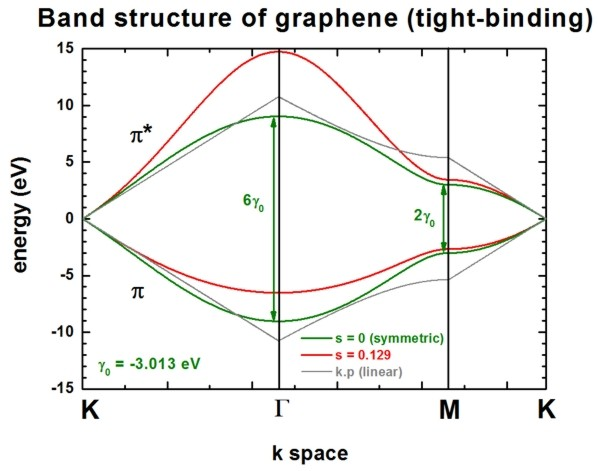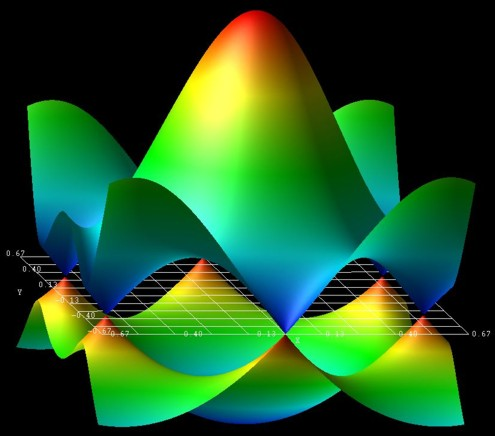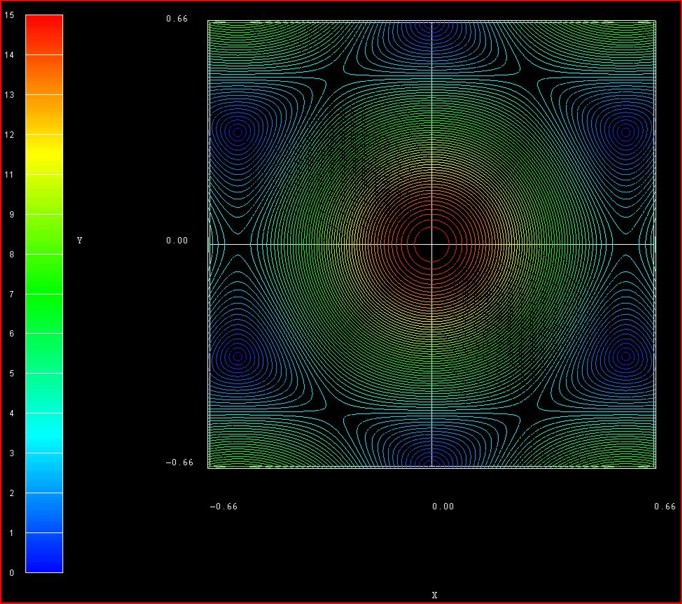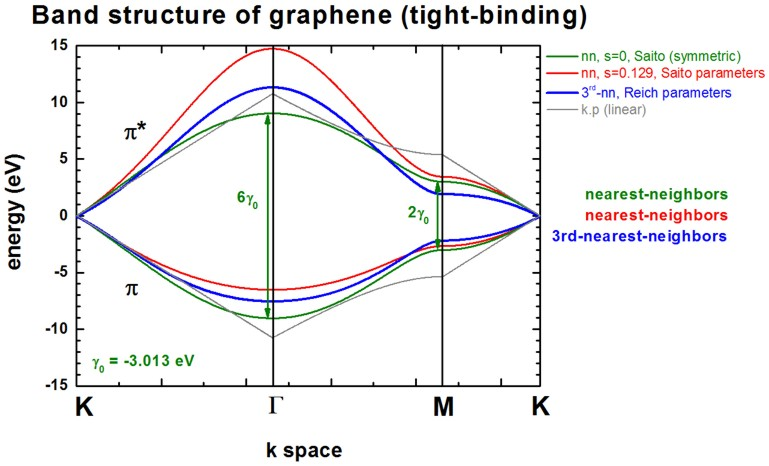nextnano.com  GUI: nextnanomat  Tool: nextnano++  Tool: nextnano³  Tool: nextnano.NEGF  Download | Search | Copyright | News | Publications  * password protected nextnano³ software1D Tight-binding graphene

# nextnano3 - Tutorial

## Tight-binding band structure of graphene

Author: Stefan Birner, Reinhard Scholz

If you are interested in the input files that are used within this tutorial, please contact stefan.birner@nextnano.de.
```-> 1D_TightBinding_graphene.in  ```

## Tight-binding band structure of graphene

### Nearest-neighbor tight-binding approximation

In this tutorial we calculate the bulk band structure of graphene which is a two-dimensional crystal (i.e. a monolayer of graphite) using a standard tight-binding approach.

For more details, see for example the article

Optical Properties and Raman Spectroscopy of Carbon Nanotubes
R. Saito, H. Kataura

in

Carbon Nanotubes - Synthesis, Structure, Properties, and Applications
M.S. Dresselhaus, G. Dresselhaus, P. Avouris (Eds.)
Topics in Applied Physics, Vol. 80, Springer (2001)

The following figure shows the conduction band pi* (upper part) and valence band pi (lower part) of graphene along special high-symmetry directions in the two-dimensional hexagonal Brillouin zone (k space).

The high symmetry points that are used in this graph (from left to right) are:

• K:          k = (kx,ky) = (   0     , 2/3 ) 2 pi/a
• Gamma: k = (kx,ky) = (   0     ,  0   )
• M:         k = (kx,ky) = ( 1/31/2 ,  0   ) 2 pi/a
• K':         k = (kx,ky) = ( 1/31/2 , 1/3 ) 2 pi/aTwo lines correspond to the case where the s0 parameter is set to zero, i.e. in that case the dispersion of both pi* and pi is the same (apart from the sign, i.e. they are symmetric with respect to the Fermi level EF = 0 eV). In this case the splitting energy at Gamma is three times as large as at the M point:

• splitting at Gamma: 6 gamma0  (for s0 = 0)
• splitting at M:          2 gamma0  (for s0 = 0)

Two lines correspond to the case where s0 = 0.129. pi* and pi are then nonsymmetric and are close to calculations from first principles and experimental data.

The data points are contained in this file:``` TightBinding/BandStructureGraphene.dat ```The first column contains integers which refer to the x axis (i.e. numbering of k points), the second column contains the eigenvalue of pi* in units of` [eV] `(conduction band), the third column contains the eigenvalue of pi in units of` [eV] `(valence band).
The file` TightBinding/k_vectors.dat `contains information about which integer corresponds to which kx and ky value.

The general formula for these lines read:

E+,`-` = [ E2p `-`+ gamma0 w(k) ] / [ 1 `-`+ s0 w(k) ]

The parameters can be specified in the input file:

```   \$numeric-control     ...     ```(nearest-neighbor approximation, i.e. 3 parameters)```     tight-binding-parameters =  0d0     ! [eV] ```E2p: site energy of the 2pz atomic orbital (orbital energy)
```                               -3.013d0 ! [eV] ```gamma0: C-C transfer energy  (usually it holds: `-`3 eV < gamma0 < `-`2.5 eV)
```                                0.129d0 ! []   ```s0 = 0.129: denotes the overlap of the electronic wave function on adjacent sites
```                             !  0d0     ! []   ```s0 = 0:       denotes the overlap of the electronic wave function on adjacent sites
```                                        !      ```(usually it holds: s0 < 0.1. Since this value is small, very often, it is neglected.)

Then there are two lines that are linear around the K point (k.p approximation or linear expansion). Their linear dispersion is independent of the parameter s0. Thus for small values of k (i.e. with respect to the K point), the energy dispersion can be approximated by a linear dispersion relation.

E(k) = E2p +`-` hbar vF | k | = E2p +`-` 31/2 gamma0 k a / 2

where a is the lattice constant of graphene (a = 0.24612 nm) and
the Fermi velocity of the charge carriers is given by vF = 31/2 | gamma0 | a / (2 hbar) ~= 0.98 * 106 m/s ~= 0.003 c
where c is the velocity of light.

These data points are contained in this file:``` TightBinding/BandStructureGraphene_kp.dat```

At the K point, the band gap is zero.

The following figure shows the energy dispersion E(kx,ky) of graphene for s0 = 0.129.
At the K points, the band gap is zero.
The point in the middle is the Gamma point.
kx is from [`-`2/3,2/3] 2 pi/a, the same holds for ky.
These data points are contained in the files:``` - TightBinding/BandStructureGraphene_cb.vtr - TightBinding/BandStructureGraphene_vb.vtr```The figure has been generated using the AVS/Express software.

The following figure shows the contour plot of the energy dispersion E(kx,ky) of graphene for s0 = 0 for the conduction band.
(Note that the valence band dispersion is identical, apart from the sign, for s0 = 0.)
These data points are contained in the file:``` TightBinding/BandStructureGraphene_cb.vtr```The following figure shows the contour plot of the energy dispersion E(kx,ky) of graphene for s0 = 0.129 for the conduction band.
These data points are contained in the file:``` TightBinding/BandStructureGraphene_cb.vtr ```The following figure shows the contour plot of the energy dispersion E(kx,ky) of graphene for s0 = 0.129 for the valence band.
These data points are contained in the file:``` TightBinding/BandStructureGraphene_vb.vtr ```nextnanomat screenshot for the conduction band dispersion E(kx,ky). The six dark areas correspong to the Dirac points in graphene.### Output options

``` !---------------------------------------------------------------------------! \$output-kp-data  ...  bulk-kp-dispersion-3D = yes  ! ```In this case, this refers to the bulk 2D tight-binding energy dispersion E(kx,ky).```                               ! ```If``` yes```, then the two-dimensional energy dispersion E(kx,ky) is written out.```  ```

```\$tighten  destination-directory = TightBinding/  !---------------------------------------------------------------------------!  ! This parameter defines the resolution of the E(kx,ky) energy dispersion.  ! E.g. if 'number-of-k-points = 100' then the kx gridding has a total of  ! [-100,...,0,...,100] grid points,  ! i.e. a total of 2 * 100 + 1 = 201 grid points along the kx direction.  ! The same applies for ky direction.  !---------------------------------------------------------------------------!  number-of-k-points = 100                                                    ! ==> 2 * n + 1  ```

### Third-nearest-neighbor tight-binding approximation

The following figure shows the band structure of graphene.
All lines are identical to the ones shown already above with the exception of the blue lines which is the third-nearest-neighbor tight-binding approximation.The third-nearest-neighbor tight-binding approximation is described in the following paper:

[Reich]
Tight-binding description of graphene
S. Reich, J. Maultzsch, C. Thomsen, P. Ordejon
Physical Review B 66, 035412 (2002)

The following parameters have been used:

`    `(third-nearest-neighbor approximation, i.e. 7 parameters)```     tight-binding-parameters = -0.28d0  ! [eV] ```E2p: site energy of the 2pz atomic orbital (orbital energy)
```                               -2.97d0  ! [eV] ```gamma0: C-C transfer energy (nearest-neighbor, nn)
```                                0.073d0 ! []   ```s0: denotes the overlap of the electronic wave function on adjacent sites (nn)
```                               -0.073d0 ! [eV] ```gamma1: (2nd-nn)
```                                0.018d0 ! []   ```s1:          (2nd-nn)
```                               -0.33d0  ! [eV] ```gamma2: (3rd-nn)
```                                0.026d0 ! []   ```s2:          (3rd-nn)
`    `(The parameters are taken from Reich et al. Note that the band gap at the K point is not exactly zero when using these parameters.)

### More options...

Inside the code several options, i.e. several algorihms, exist to setup the tight-binding Hamiltonian:

```tight-binding-method = bulk-graphene-Saito-nn       !     nn = ```       nearest-neighbor```                      = bulk-graphene-Scholz-nn      !     nn = ```       nearest-neighbor```                      = bulk-graphene-Scholz-3rd-nn  ! 3rd-nn = ```third-nearest-neighbor```                      = bulk-graphene-Reich-3rd-nn   ! 3rd-nn = ```third-nearest-neighbor

Special option for third-nearest-neighbor tight-binding approximation in graphene:

```tight-binding-calculate-parameter = no     ! ```(default) use 7 parameters (E2p,gamma0,gamma1,gamma2,s0,s1,s2)```                                   = E2p    ! ```            use 6 parameters (       gamma0,gamma1,gamma2,s0,s1,s2)```                                   = gamma1 ! ```            use 6 parameters (E2p,gamma0,             gamma2,s0,s1,s2)
E2p or gamma1 can be calculated internally in order to force E(K) = 0 eV where K is the K point in the Brillouin zone. In that case, only 6 parameters are used (although 7 parameters have to be present in the input file). The parameter to be calculated is simply ignored inside the code.

The k space resolution, i.e. the number of grid points on the axis of these plots can be adjusted.

```  \$tighten    calculate-tight-binding-tighten = no              !    destination-directory           = TightBinding/    number-of-k-points              = 50              ! ```This corresponds to` 50 `k points between the Gamma point and the M point.```                                                      ! ```The number of k points along the other directions are scaled correspondingly.```  ```

• Please help us to improve our tutorial. Send comments to``` support [at] nextnano.com```.